Courses

# Introduction to Metal Oxide Semiconductor Field Effect Transistor (MOSFET) Electrical Engineering (EE) Notes | EduRev

## VLSI System Design

Created by: Machine Experts

## Electrical Engineering (EE) : Introduction to Metal Oxide Semiconductor Field Effect Transistor (MOSFET) Electrical Engineering (EE) Notes | EduRev

The document Introduction to Metal Oxide Semiconductor Field Effect Transistor (MOSFET) Electrical Engineering (EE) Notes | EduRev is a part of the Electrical Engineering (EE) Course VLSI System Design.
All you need of Electrical Engineering (EE) at this link: Electrical Engineering (EE)

Objectives

In this course you will learn the following

• Basic MOS Structure
• Types of MOSFET
• MOSFET I-V Modelling

3.1 Basic MOSFET Structure

In the introduction to a system, we got an overview of various levels of design, viz. Architectural level design, Program level design, Functional level design and Logic level design. However we can't understand the levels of design unless we are exposed to the basics of operation of the devices currently used to realize the logic circuits, viz., MOSFET (Metal Oxide Semiconductor Field Effect Transistor). So in this section, we'll study the basic structure of MOSFET.

The cross-sectional and top/bottom view of MOSFET are as in figures 3.11 and 3.12 given below :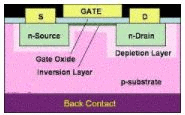Fig 3.11: Cross-sectional view of MOSFET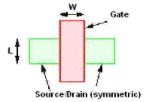Fig 3.12: Top/Bottom View of MOSFET

An n-type MOSFET consists of a source and a drain, two highly conducting n-type semiconductor regions which are separated from the p-type substrate by reverse-biased p-n diodes. A metal or poly crystalline gate covers the region between the source and drain, but is isolated from the semiconductor by the gate oxide.

3.2 Types of MOSFET

MOSFETs are divided into two types viz. p-MOSFET and n-MOSFET depending upon its type of source and drain.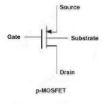Fig. 3.21: p-MOSFET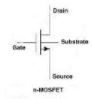Fig. 3.22: n-MOSFET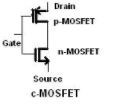Fig. 3.23: c-MOSFET

The combination of a n-MOSFET and a p-MOSFET (as shown in figure 3.21) is called cMOSFET which is the mostly used as MOSFET transistor. We will look at it in more detail later.

3.3 MOSFET I-V Modelling

We are interested in finding the output characteristics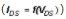and the transfer charcteristics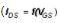of the MOSFET. In other words, we can find out both if we can formulate a mathematical equation of the form :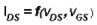Intutively, we can say that voltage level specifications and the material parameters cannot be altered by designers. So the only tools in the designer's hands with which he/she can improve the performance of the device are its dimensions, W and L (shown in top view of MOSFET fig 2). In fact, the most important parameter in the device simulations is ratio of W and L.

The equations governing the output and transfer characteristics of an n-MOSFET and p-MOSFET are :

p-MOSFET: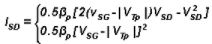Linear  Saturation

n-MOSFET: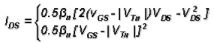Linear  Saturation

The output characteristics plotted for few fixed values of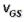for p-MOSFET and n-MOSFET are shown next :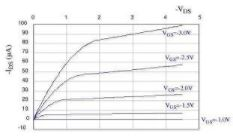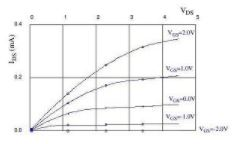Fig 3.31: p-MOSFET                                            Fig 3.32: n-MOSFET

The transfer characteristics of both p-MOSFET and n-MOSFET are plotted for a fixed value of VDS as shown next :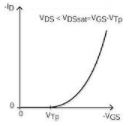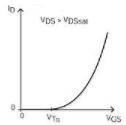Fig 3.33: p-MOSFET            Fig 3.34: n- MOSFET

Note: From now onwards in the lectures, we will symbolize MOSFET by MOS.

3.4 C-V Characteristics of a MOS Capacitor

As we have seen earlier, there is an oxide layer below Gate terminal. Since oxide is a very good insulator, it contributes to an oxide capacitance in the circuit. Normally, the capacitance value of a capacitor doesn't change with values of voltage applied across its terminals. However, this is not the case with MOS capacitor. We find that the capacitance of MOS capacitor changes its value with the variation in Gate voltage. This is because application of gate voltage results in the band bending of silicon substrate and hence variation in charge concentration at Si-SiO2 interface. Also we can see (from fig.3.42 ) that the C.VG curve splits into two (reason will be explained later), after a certain voltage, depending upon the frequency (high or low) of AC voltage applied at the gate. This voltage is called the threshold voltage (Vth) of MOS capacitor.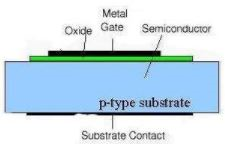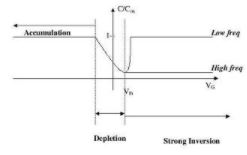Fig 3.41: Cross-section view of MOS Capacitor        Fig 3.42: C.VG plot of MOS Capacitor

Offer running on EduRev: Apply code STAYHOME200 to get INR 200 off on our premium plan EduRev Infinity!

35 docs|28 tests

,

,

,

,

,

,

,

,

,

,

,

,

,

,

,

,

,

,

,

,

,

;